### The Rosenbrock Problem

You can use parameters to produce a clear formulation of a problem. Consider the Rosenbrock problem,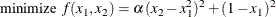where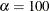is a parameter (constant),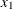and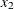are optimization variables (whose values are to be determined), and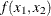is an objective function.

Here is a PROC OPTMODEL program that solves the Rosenbrock problem:

proc optmodel;
number alpha = 100; /* declare parameter */
var x {1..2};       /* declare variables */
/* objective function */
min f = alpha*(x - x**2)**2 +
(1 - x)**2;
/* now run the solver */
solve;

print x;
quit;


The PROC OPTMODEL output is shown in Figure 5.3.

Figure 5.3: Rosenbrock Function Results

The OPTMODEL Procedure

Problem Summary
Objective Sense Minimization
Objective Function f
Objective Type Nonlinear

Number of Variables 2
Bounded Above 0
Bounded Below 0
Bounded Below and Above 0
Free 2
Fixed 0

Number of Constraints 0

Performance Information
Execution Mode Single-Machine

Solution Summary
Solver NLP
Algorithm Interior Point
Objective Function f
Solution Status Optimal
Objective Value 8.206033E-23

Optimality Error 9.707102E-11
Infeasibility 0

Iterations 14
Presolve Time 0.00
Solution Time 0.01

 x
1 1
2 1